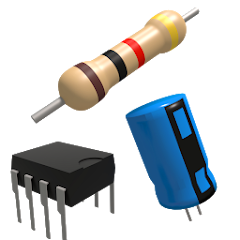# Electronics Toolkit

4.4
11.6K reviews
1M+Everyone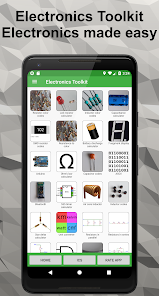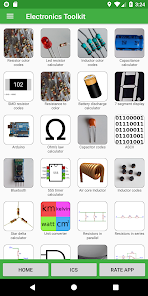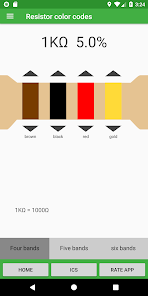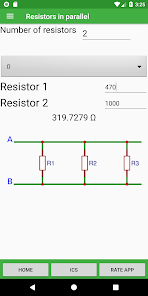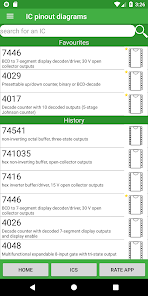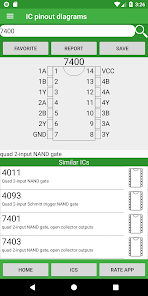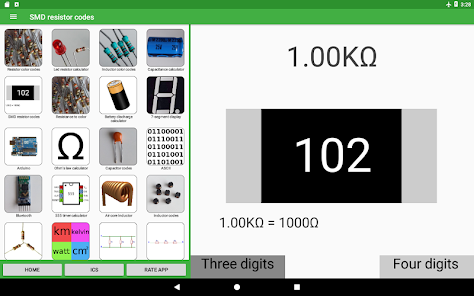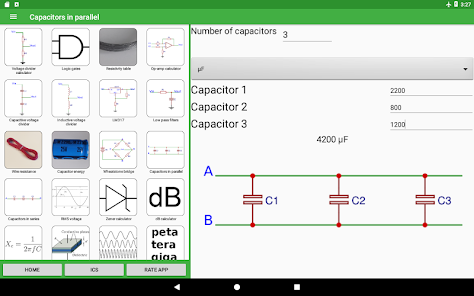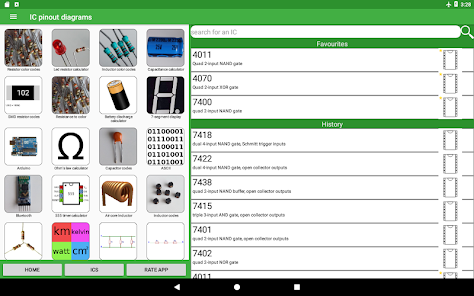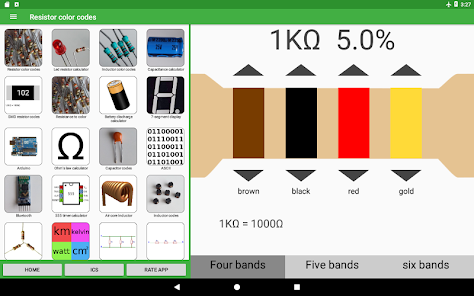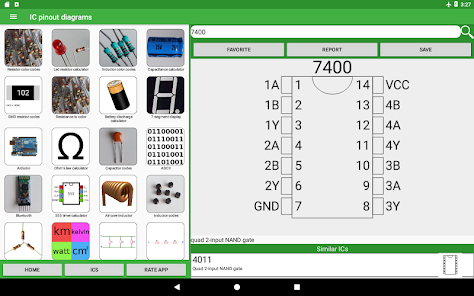Electronics Toolkit is a helpful app with dozens of tools, calculators and references made for electronic engineers, students and hobbyists.

Calculators:
• Resistor color codes - calculate the resistance of resistors by selecting the colors of the bands

• SMD resistor codes - calculate the resistance of SMD resistors by entering the number

• LED resistor calculator - calculate the needed resistance to connect en LED to a power source

• Parallel resistors - calculate the resistance of resistors in parallel

• Voltage divider - calculate the output voltage of a voltage divider

• Series resistors - calculate the resistance of resistors in series

• Ohm's law - calculate the voltage, current of resistance by entering the other two

• Capacitance calculator - calculate the capacitance, voltage or charge by entering the other two

• Battery discharge - calculate the time it takes to discharge a battery

• Inductor color codes - calculate the inductance of inductors by selecting the colors of the bands

• Parallel capacitors - calculate the capacitance of capacitors in parallel

• Series capacitors - calculate the capacitance of capacitors in series

• Unit converter - unit converter for length, temperature, area, volume, weight, time, angle, power and base

• Op-amp calculator - calculate the output voltage of non-inverting, inverting, summing and differential opamps

• Wheatstone bridge - calculate the resistance of one resistor in a balanced bridge or calculate the output voltage

• Inductor codes - calculate the inductance of inductors by entering the number

• Capacitor codes - calculate the capacitance of capacitors by entering the number

• DAC and ADC calculator - calculate the output of digital-analog and analog-digital converters

• Wavelength frequency calculator - calculate the frequency or wavelength of a wave

• SI prefixes - convert numbers with SI prefixes

• Capacitor energy - calculate the energy in an capacitor

• Slew rate calculator - calculate the slew rate

• Star delta transformation - calculate the resistors in a star delta transformation

• Zener calculator - calculate the resistance of the resistor and voltage of the zener

• Air core inductor calculator - calculate the inductance and wire length of an air core inductor

• 555 timer calculator - calculate the frequency, period, duty cycle, high time and low time of a popular 555 timer circuit

• Plate capacitor calculator - calculate the capacity of a plate capacitor

• Resistance to color code calculator - calculate the colors on the resistor by entering the resistance

• LM317 - calculate the output voltage of an LM317

• Low pass filters

• Wire resistance - calculate the resistance of electrical wire

• RMS voltage

• Decibel calculator

•Reactance

Tables:
• Logic gates - truth table of the 7 logic gates with interactive buttons

• 7-segment display - interactive display that you can change by clicking on one of the segments or by clicking on a button to show a hexadecimal character

• ASCII - decimal, hexadecimal, binary, octal and char ASCII table

• Resistivity - table with the resistivity of common metals at 293K

• Arduino pinout

• Pinout diagrams of 4000 and 7400 series ICs

Other:
• Bluetooth - connect to a bluetooth module like the HC-05 to talk with an arduino or other microcontroller with the terminal, button and slider modes

PERMISSIONS
• read the contents of your USB storage && modify or delete the contents of your USB storage - used to save images of IC pinout diagrams

• receive data from Internet && view network connections && full network access - used to load the IC data list and to collect statistics with Google Firebase

• pair with Bluetooth devices && access Bluetooth settings - used to connect with bluetooth devices

• prevent device from sleeping - prevents bluetooth devices from disconnecting
Updated on
Mar 22, 2021

## Data safetyNo information available

## Ratings and reviews

4.4
11.1K reviews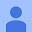April 7, 2021
To be honest, just buy it. This app is worth it. Espically for an eletroinic engineering major. Or even if you just are in a electroincs class this really helps understand and solve problems.. Its has everything you need. I used it to repair a led connected to gen transfer switch. No ads when you buy it.. Best things to do is The program you use, put it in your favorites..
94 people found this review helpful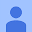November 30, 2019
The app is nice for electricians but for curious people like me (with no electric experience whatsoever) it would be nice to have explanations, definitions and conversions, like explaining which number each band represents in a resistor. Thanks.
32 people found this review helpful